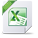# Power BI Exchange

View Only

## Help needed for Calculations in Power BI Matrix

• #### 1.  Help needed for Calculations in Power BI Matrix

Posted 26 days ago
Edited by Ulrich Schumann 26 days ago
Hi all,

I would like to create a Matrix in Power BI where %-Values are calculated per Row and per Category/Sub-Category. This is an easy task in Excel, but I struggle to implement this in Power BI Matrix visualization.Any ideas are welcome. Example file is attached.

Solved: My mistake was to put the formula into calculated column (which leads to overall percentage) instead of using a measure.

------------------------------
Ulrich Schumann
------------------------------

Attachment(s)Example Matrix_Calc.xlsx   16 KB 1 version